• 2.2纯随机性检验 白噪声序列 随机序列也称为白噪声序列，满足如下两条性质(1)EXt=μ,∀t∈T(1)EX_t=\mu,\forall t\in T(1)EXt​=μ,∀t∈T(2)γ(t,s)={σ2,t=s0,t=s,∀t,s∈T(2)\gamma(t,s)= \begin {case...

本文为本科课程笔记 原创思路是老师的~~ 不知道会不会侵犯啥权益，所以声明一下~~ 用作记录~~

2.2纯随机性检验
白噪声序列
纯随机序列也称为白噪声序列，满足如下两条性质

(

1

)

E

X

t

=

μ

,

∀

t

∈

T

(1)EX_t=\mu,\forall t\in T

(

2

)

γ

(

t

,

s

)

=

{

σ

2

,

t

=

s

0

,

t

=

s

,

∀

t

,

s

∈

T

(2)\gamma(t,s)= \begin {cases} \sigma^2, &t=s \\ 0,&t=s \end{cases}, \forall t,s\in T

纯随机性：各序列值之间没有任何相关关系，即为“没有记忆”的序列

γ

(

k

)

=

0

,

∀

k

≠

0

\gamma(k)=0,\forall k \neq 0

方差齐性：根据马尔可夫定理，只有方差齐性假定成立时，用最小二乘法得到的未知参数估计值才是准确的、有效的

D

(

X

t

)

=

γ

(

0

)

=

σ

2

D(X_t)=\gamma(0)=\sigma^2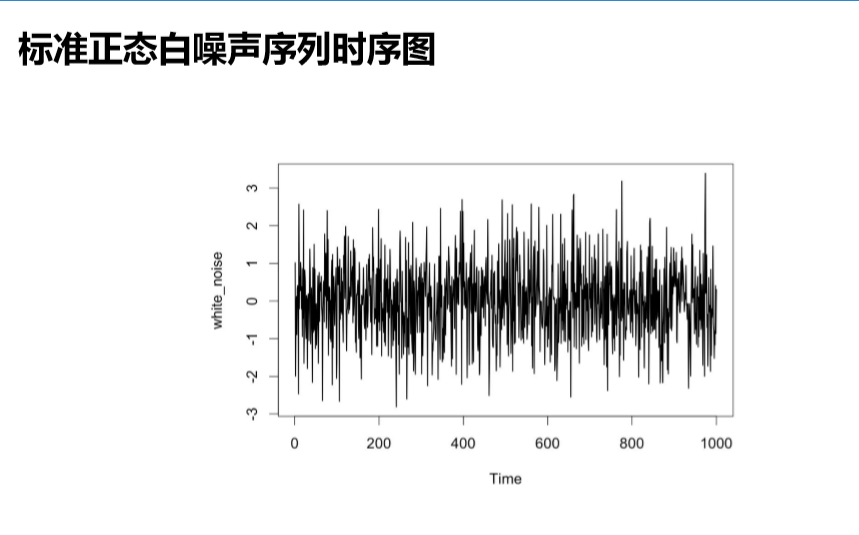纯随机性检验
检验原理：Barlett定理
如果一个时间序列是纯随机的，得到一个观察期数为n的观察序列，那么该序列的延迟非零期的样本自相关系数将近似服从均值为0，方差为序列观察期数倒数的正态分布。

ρ

k

^

∼

N

(

0

,

1

n

)

,

∀

k

≠

0

\hat{\rho_k} \sim N(0,\frac{1}{n} ),\forall k\neq 0

假设条件：
原假设：延迟期数小于或等于m期的序列值之间相互独立

H

0

:

ρ

1

=

ρ

2

=

⋯

=

ρ

m

=

0

,

∀

m

≥

1

H_0:\rho _1= \rho _2=\cdots=\rho_m=0,\forall m \ge1

备择假设：延迟期数小于或等于m期的序列值之间有相关性

H

1

:

至

少

存

在

某

个

ρ

k

≠

0

,

∀

m

≥

1

,

k

≤

m

H_1:至少存在某个\rho_k\neq0,\forall m \ge1,k\le m

检验统计量
Q统计量

Q

=

n

∑

k

=

1

m

ρ

k

2

^

∼

χ

2

(

m

)

Q=n\sum_{k=1}^m \hat{\rho_k^2}\sim \chi^2(m)

LBQ统计量

L

B

=

n

(

n

+

2

)

∑

k

=

1

m

(

ρ

k

2

^

n

−

k

)

∼

χ

2

(

m

)

LB=n(n+2)\sum_{k=1}^m(\frac{\hat{\rho_k^2}}{n-k})\sim \chi^2(m)

判别原则
当检验统计量大于

χ

1

−

α

2

(

m

)

\chi ^2_{1-\alpha}(m)

分位点，或该统计量的

p

p

值小于

α

\alpha

时，则可以以

1

−

α

1-\alpha

的置信水平拒绝原假设，认为该序列为非白噪声序列. 
展开全文• 用python处理时间序列数据，检验平稳性跟纯随机性
用python处理时间序列数据，检验平稳性跟纯随机性
from statsmodels.tsa.stattools import adfuller as adf
from statsmodels.graphics.tsaplots import plot_acf, plot_pacf
import pandas as pd
import numpy as np

!pip install statsmodels

Requirement already satisfied: statsmodels in c:\programdata\anaconda3\lib\site-packages (0.11.0)
Requirement already satisfied: numpy>=1.14 in c:\programdata\anaconda3\lib\site-packages (from statsmodels) (1.18.1)
Requirement already satisfied: scipy>=1.0 in c:\programdata\anaconda3\lib\site-packages (from statsmodels) (1.4.1)
Requirement already satisfied: pandas>=0.21 in c:\programdata\anaconda3\lib\site-packages (from statsmodels) (1.0.1)
Requirement already satisfied: patsy>=0.5 in c:\programdata\anaconda3\lib\site-packages (from statsmodels) (0.5.1)
Requirement already satisfied: pytz>=2017.2 in c:\programdata\anaconda3\lib\site-packages (from pandas>=0.21->statsmodels) (2019.3)
Requirement already satisfied: python-dateutil>=2.6.1 in c:\programdata\anaconda3\lib\site-packages (from pandas>=0.21->statsmodels) (2.8.1)
Requirement already satisfied: six in c:\programdata\anaconda3\lib\site-packages (from patsy>=0.5->statsmodels) (1.14.0)

data=pd.read_excel('./data.xls',encoding='utf-8')
data


timewc(误差随机项)xt1xt2xt3011.741.0000001.0001.00012-0.700.8000000.8000.80023-1.282.6500001.4401.430340.437.4400005.0944.060450.2413.80400010.2045.645..................95961.55739.0866856908.698146.49096970.07748.3220117056.498147.3459798-0.73756.3832077205.072148.14098990.66765.1299247356.450150.72599100-0.44773.1879547508.420151.515
100 rows × 5 columns
对X1做平稳性检验
xt1=data.xt1
pd.Series(dftest[0:4],index=['Test Statistic','p-value','#Lags Used','Number of Observations Used'])
#p值高达0.9几

Test Statistic                  0.678947
p-value                         0.989408
#Lags Used                      1.000000
Number of Observations Used    98.000000
dtype: float64

xt1.plot()

<matplotlib.axes._subplots.AxesSubplot at 0x238faff6208>[output_6_1.png)]
#一阶差分
xt1_1 = xt1.diff(1)
xt1_1.plot()

<matplotlib.axes._subplots.AxesSubplot at 0x238fa6a5048>[(output_7_1.png)]
#一阶差分的单位根检验
pd.Series(dftest_1[0:4],index=['Test Statistic','p-value','#Lags Used','Number of Observations Used'])
#一阶差分后p值小于0.05,拒绝原假设（即不存在单位根，认为其已经平稳）

Test Statistic                -6.056515e+00
p-value                        1.241430e-07
#Lags Used                     0.000000e+00
Number of Observations Used    9.800000e+01
dtype: float64

dftest_1-0.05

-0.049999875856979056

#画一阶差分之后的自相关图跟偏自相关图
plot_acf(xt1_1.dropna())[(output_10_0.png)]
plot_pacf(xt1_1.dropna())[(output_11_0.png)]
X2做平稳性检验
data.xt2.plot()

<matplotlib.axes._subplots.AxesSubplot at 0x238fe204d88>(output_13_1.png)]
#做二阶差分
x2_2=data.Xt2一阶差分.diff(1)
x2_2.plot()

<matplotlib.axes._subplots.AxesSubplot at 0x238fc1d7a48>[output_14_1.png)]
#对二阶差分后的Xt2做单位根检验
#故拒绝原假设，该序列平稳

(-7.1910449486700525,
2.5032477359463947e-10,
4,
93,
{'1%': -3.502704609582561,
'5%': -2.8931578098779522,
'10%': -2.583636712914788},
260.6359245108364)

#画二阶差分后的Xt2的自相关图跟偏自相关图
plot_acf(x2_2.dropna())(output_16_0.png)]
plot_pacf(x2_2.dropna())(output_17_1.png)]
对X3做平稳性检验
data.xt3.plot()

<matplotlib.axes._subplots.AxesSubplot at 0x238fd5efc08>(output_19_1.png)]
#做一阶差分
data.xt3.diff(1).plot()

<matplotlib.axes._subplots.AxesSubplot at 0x238ff5dac88>(output_20_1.png)]
#对一阶差分后的序列做单位根检验

(-10.661639595719135,
4.391819453885797e-19,
1,
97,
{'1%': -3.4996365338407074,
'5%': -2.8918307730370025,
'10%': -2.5829283377617176},
257.9188344687909)

adf(data.xt3.diff(1).dropna())-0.05
#故拒绝原假设，该序列平稳

-0.05

#对一阶差分后的Xt3画出自相关图跟偏自相关图
plot_acf(data.xt3.diff(1).dropna())（output_23_1.png)]
plot_pacf(data.xt3.diff(1).dropna())(output_24_1.png)]


展开全文python 数据分析 numpy pandas
• 本文为本科课程整理 ...#纯随机性检验 white_noise <- rnorm(500) white_noise2 <- rnorm(500,mean = 2,sd = 0.2) white_noise <- ts(white_noise) plot(white_noise) acf(white_noise,ylim=c(-1,1))...

本文为本科课程整理 五一应该会做一个大概的纸质版的笔记整理。

#纯随机性检验
white_noise <- rnorm(500)
white_noise2 <- rnorm(500,mean = 2,sd = 0.2)
white_noise <- ts(white_noise)
plot(white_noise)
acf(white_noise,ylim=c(-1,1))

#LBQ检验
LBQ <- Box.test(white_noise,lag = 6,type = "Ljung-Box")
help(Box.test)
LBQ$p.value #for循环 for (i in 1:2) print(Box.test(white_noise,lag = 6*i)) LBQ_p <- 1:24 for(i in 1:24){ LBQ <- Box.test(white_noise,lag = 6*i) LBQ_p[i]<- LBQ$p.value
}

prop <- ts(c$prop,start = 1950) plot(prop) acf(prop,ylim=c(-1,1)) for (i in 1:2) print(Box.test(prop,lag=6*i)) 纯随机性检验 #纯随机性检验 white_noise <- rnorm(500) white_noise2 <- rnorm(500,mean = 2,sd = 0.2) white_noise <- ts(white_noise) plot(white_noise) acf(white_noise,ylim=c(-1,1)) rnorm（）：可以输入均值和标准差。不给的话默认为标准正态分布。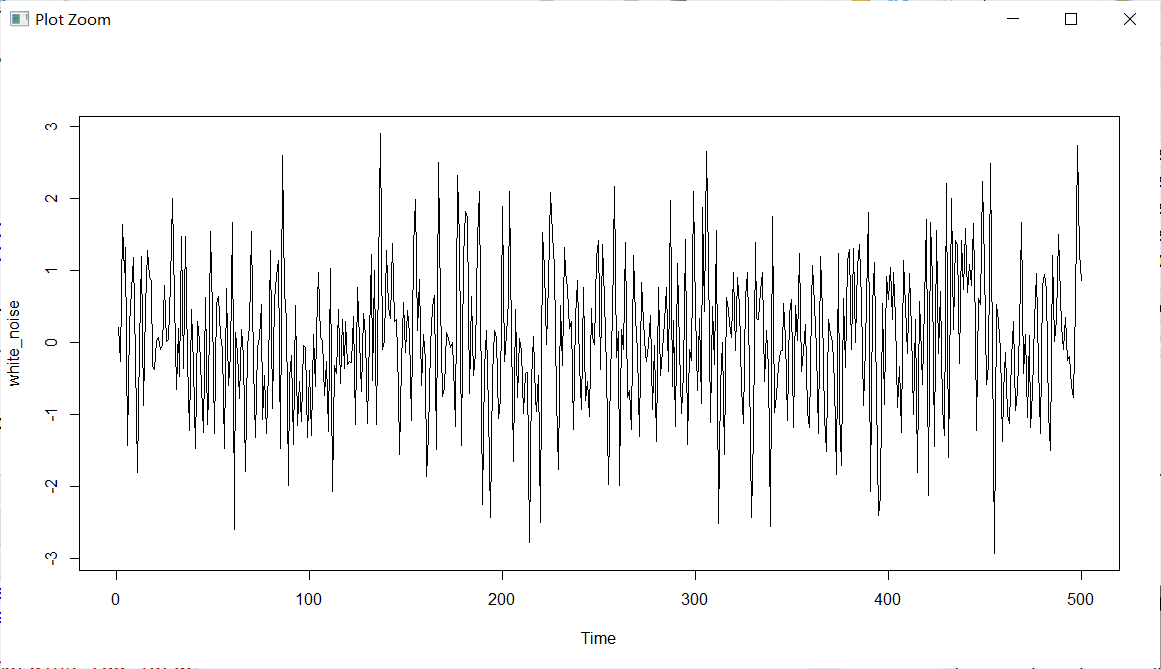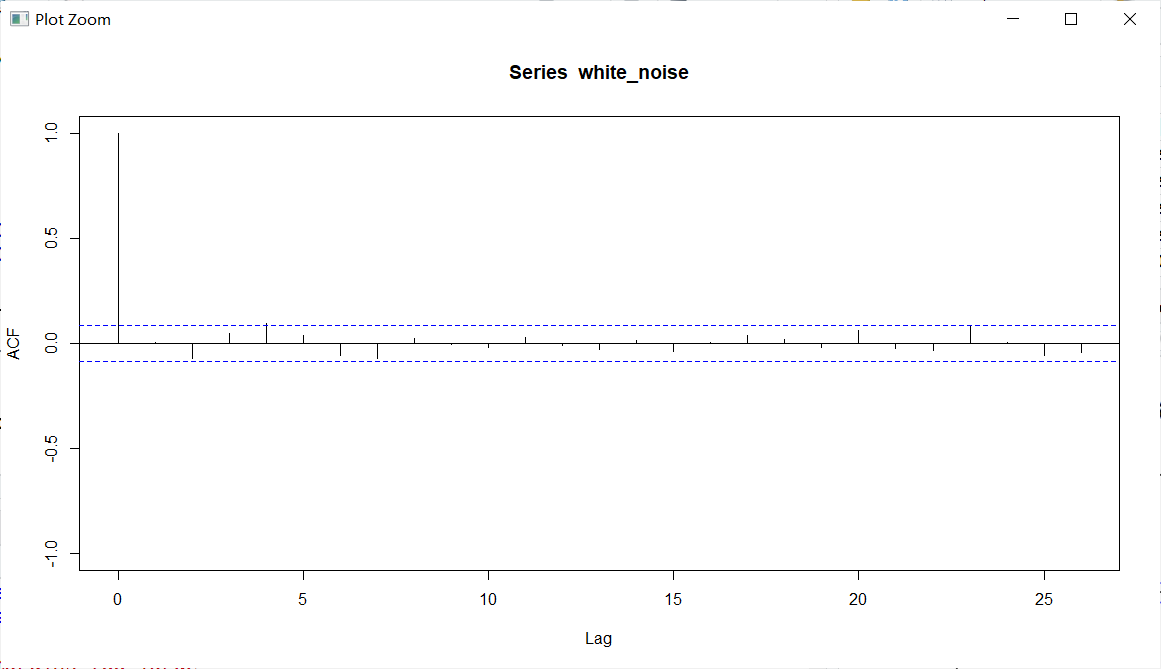LBQ检验——Box.text() #LBQ检验 LBQ <- Box.test(white_noise,lag = 6,type = "Ljung-Box") help(Box.test) LBQ$p.value
Box.test:
lag:检验的滞后阶数Q检验分为BPQ和LBQ检验。 LBQ检验效果更好一些。 type给一个LBQ检验来运行。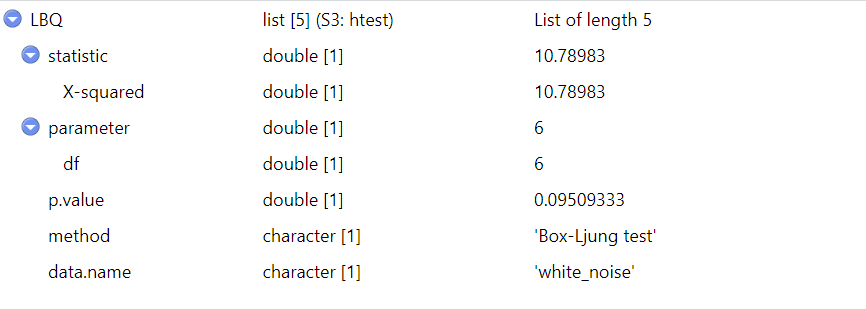在拿到一个时间序列时，先把时序图画出来，看是不是平稳的，然后对其进行平稳性检验。如果不是平稳的，就要想办法把它化成平稳的时间序列。之后需要对其进行LBQ检验。 -为什么进行纯随机性检验？ -如果它是白噪声序列，就没必要用统计的方法进行分析了。

#for循环
for (i in 1:2) print(Box.test(white_noise,lag = 6*i))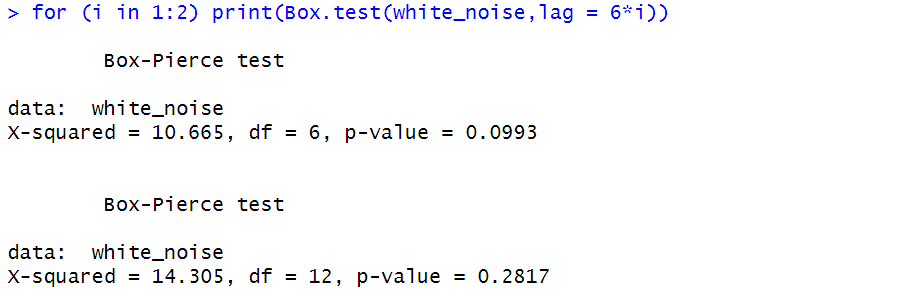LBQ_p <- 1:24
for(i in 1:24){
LBQ <- Box.test(white_noise,lag = i)
LBQ_p[i]<- LBQ$p.value }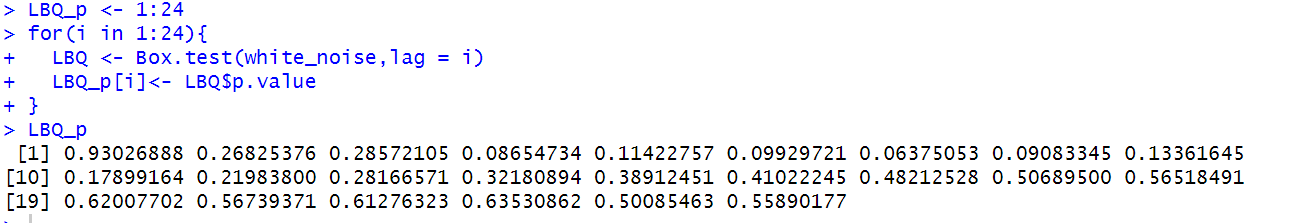教材40页例子 c<-read.csv()#教材40页 prop <- ts(c$prop,start = 1950)
plot(prop)
acf(prop,ylim=c(-1,1))

for (i in 1:2) print(Box.test(prop,lag=6*i))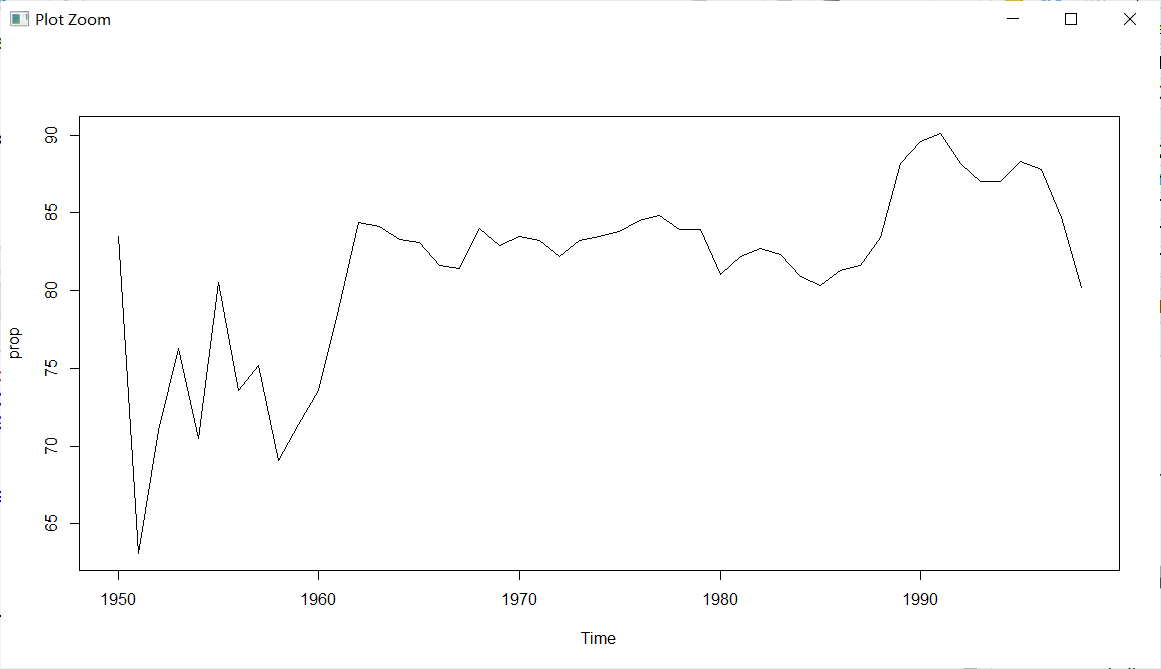没有明显趋势，还是要用ACF检验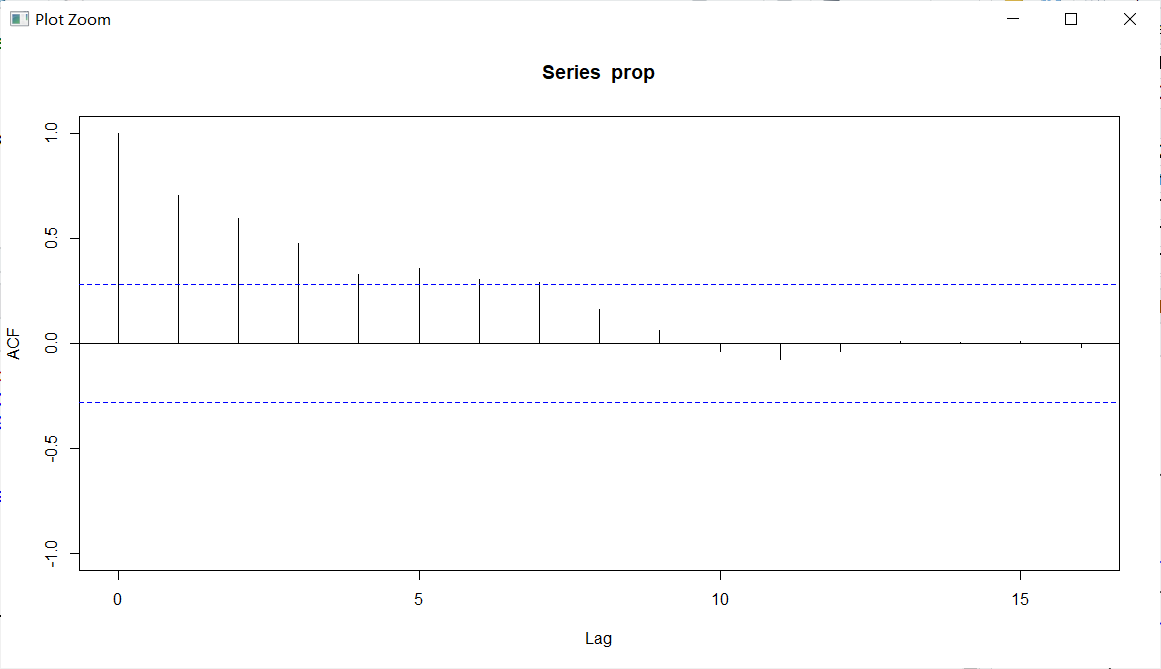通过自相关看到前面几阶显著，后面就衰减了 然后进行LBQ检验，只检验了6和12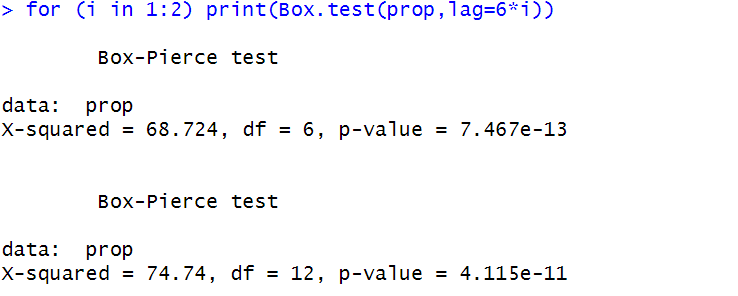发现p值都小于0.05，说明存在自相关情况，不是白噪声序列。
展开全文• 本章主要介绍进行时序分析前的预处理，即平稳性检验与纯随机性检验。 平稳性检验（描述性） 平稳性检验的方法分为描述性方法与计量性方法。前者主要指时序图检验、ACF 图检验，后者主要指 DF 检验、ADF 检验与PP...
这篇博客主要记录人大出版《应用时间序列分析》第二章的笔记。本章主要介绍进行时序分析前的预处理，即平稳性检验与纯随机性检验。
平稳性检验（描述性）
平稳性检验的方法分为描述性方法与计量性方法。前者主要指时序图检验、ACF 图检验，后者主要指 DF 检验、ADF 检验与PP检验。由于计量性方法需要 ARMA 模型的相关知识，这篇博客仅仅介绍描述性方法。
时序图检验
时序图检验即是通过观察时序图来判断时间序列是否平稳。Python 中画时序图的代码如下：
import pandas as pd
import matplotlib.pyplot as plt
data = pd.DataFrame({'2010-01-01': 10.00, '2010-01-02': 13.00, '2010-01-04': 13.50, '2010-01-05': 13.50, '2010-01-06': 14.50, '2010-01-07': 16.00, '2010-01-08': 20.50, '2010-01-10': 24.50, '2010-01-11': 27.50, '2010-01-12': 30.50}, index=['price'])
data = data.T
data['price'].plot()
plt.show()
作图结果如下：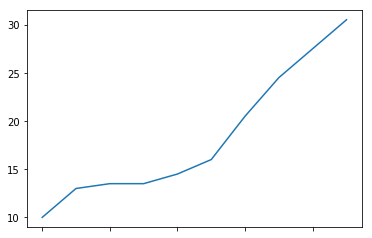其实就是使用 pandas 中内置的折线图。
具体如何通过时序图来检验平稳性呢？平稳时序定义要求均值、方差为常数，协方差仅仅与时间间隔相关。从定义入手，时序图满足以下任一条件的不是平稳时序：
时序存在明显的趋势，即均值不为常数时序存在集群效应，即某段时间的波动幅度较其它时段明显较大或者较小，即方差不为常数
下图为集群效应的一个例子：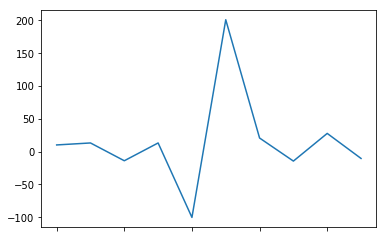时序中间时段的波动幅度明显大于两端，可以认为时序存在集群效应，为非平稳时序。集群效应在金融时间序列中往往十分常见。
ACF图检验
上篇博客中介绍了自协方差函数的定义与作图方法，链接：https://blog.csdn.net/weixin_44607126/article/details/89086035
平稳时序往往仅具有短期自相关性，长期的 ACF 会振荡随机趋近于0。因此，当 ACF 图不满足这一条件时，可以认为时序非平稳。如何理解振荡与随机呢？下面给出几个 ACF 图检验的例子。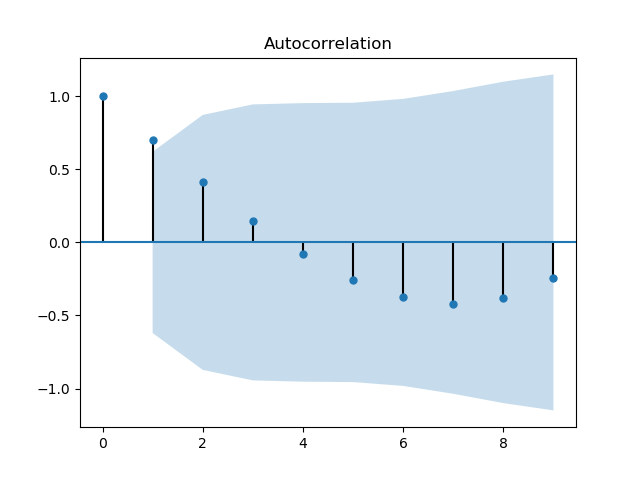上图在 k >= 2 时就已经趋于 0 了，但是并没有满足随机趋于 0 的条件，该图中的 ACF(k) 先连续4项正值，然后连续6项负值，整个图形呈现出倒三角的形状，这意味着时序中存在明显的趋势，为非平稳时序。事实上，这是一条单增时序的 ACF 图。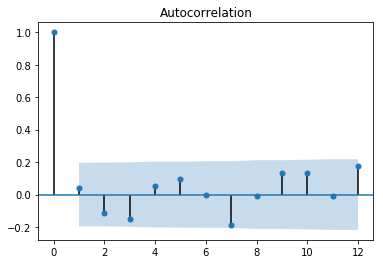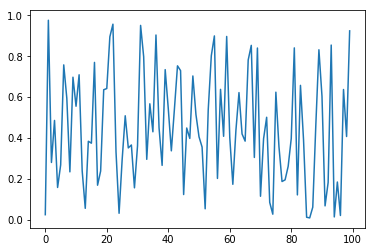上图是平稳时序的 ACF 图的一个例子，可以看到 ACF(k) 没有出现规律性，振荡随机趋近于0，可以认为时序平稳。
总结来看，当 ACF 图满足下列任一条件时，可以认为时序为非平稳时序：
ACF 图正项与负项连续交替出现，呈现倒三角形，意味着时序中存在趋势ACF 图拖尾，即当 k 很大时仍有 ACF(k) 显著
描述性检验方法的注意事项
无论是时序图还是 ACF 图，使用它们作为检验方法时都具有较强的主观性，没有引入客观的统计量。因此，时序图与 ACF 图仅能用来排除非平稳时序，不能用来判断一个时序是否是平稳时序！即描述性检验方法仅仅是为计量性检验方法作一个初步筛选，如果时序没有通过描述性检验方法，就不需要进行计量性检验了；而即使时序通过了描述性检验方法，仍需要进行计量性检验来进一步确认时序为平稳时序。
纯随机性检验
在进行时序分析之前，我们需要确认这个时序不是一个单纯的噪音，而是蕴含着可以用模型描述的信息。因此需要对时序进行纯随机性检验。
白噪声的定义
我们称满足下列条件的时序为白噪声（纯随机时序）：

白噪声满足均值与方差均为常数，且不同时刻上的随机变量不相关。显然，白噪声是一种平稳时序。
从白噪声的定义中可以看出，对于白噪声时序，历史数据不能提供关于未来的信息，此时建立时序模型将会是徒劳的。因此在蚝时序建模前需要进行白噪声检验。同时，白噪声还可以用来判断时序模型总体的显著性，当模型残差是白噪声序列时，就可以认为模型已经充分提取了时序信息。
Barlett定理
如果一个时序是纯随机的，得到一个观察期数为 n 的观察序列，那么该序列的延迟非零期样本自相关系数近似服从 的正态分布。
仅仅想在应用层面理解时序则不必深究上述定理。
BP检验
根据 Barlett 定理，可以构建 Q 统计量：
则有 ，其中 m 为指定最大延迟期数， 为 k 期延迟样本自相关系数。原假设为时序是白噪声序列，当 Q 大于单侧检验临界值时认为时序不是白噪声序列。
LB检验
LB 检验是在 BP 检验的基础上进行了一些修正，使其更适用于小样本。LB 统计量表达式为：
在实际应用中通常使用 LB 检验。
在python中进行 LB 检验的代码如下：
from statsmodels.stats.diagnostic import acorr_ljungbox
print(acorr_ljungbox(np.random.rand(100), lags=[6, 12]))
lags 即上面公式中的m ， 通常取 6 与 12。
输出如下：
(array([2.02502998, 8.28247283]), array([0.91738225, 0.76268484]))
第一个 array 是 LB 统计量的数值，第二个 array 是 p 值。当 p 值小于显著性水平时认为时序不是白噪声。
总结
在进行正式的时序建模前需要检验时序的平稳性与纯随机性平稳性检验可以通过时序图、ACF 图等描述性方法，但依然要使用 ADF 检验、PP 检验来最终判断纯随机性检验可以使用 BP 统计量与 LB 统计量，通常使用 LB 统计量
误区
仅仅通过时序图与 ACF 图就断定一个时序是平稳时序：时序图与 ACF 图仅仅只能用于判断非平稳时序，不能用于判断平稳时序不画时序图与 ACF 图，直接对时序进行 ADF 检验与 PP 检验：描述统计是必不可少的步骤，通过时序图与 ACF 图可以清楚看出时序的趋势性与周期性在正式建模之前不检验时序的纯随机性，认为仅仅检验时序的平稳性就足够了：白噪声时序也是平稳序列，但是没有分析的价值在正式建模之后不检验残差序列的纯随机性：残差序列的纯随机性检验有点类似于多元回归中的 F 检验，检验的是模型总体的显著性水平
展开全文平稳性检验 python
•     对于一个时间序列，在进行建模之前，首先需要进行平稳性检验和纯随机性检验，然后根据检验的结果再选择适合的模型。在讲解平稳性和随机性的定义之前，我们先介绍一下时间序列中常用的几个特征统计量。 2.1...
• 三、纯随机性检验 Box.test（x, type= ，lag= ）  x：变量名  type：检验统计量类型。type=“Box-Pierce”输出白噪声检验的Q统计量。该统计量为系统默认输出结果。type=“Ljung-Box”输出白噪声检验的...
• 回复悟得：时间序列(从左到右)3.1 3.3 3.1 2.6 4.4 3.6 3.6 1.8 2 1 2.9 4.5 0.6 1.1 1.2 3.9 3.7 2....
• 本文档通过案例的形式展示了平稳性检验和纯随机性检验的完整过程、结果及具体分析，能让初学者更好的理解和掌握检验的真正作用，使知识更加融汇贯通。
• 序列的平稳性与纯随机性检验，模型的有效性，参数的显著性，最优模型准则AIC,SBC data <- scan() 126.4 82.4 78.1 51.1 90.9 76.2 104.5 87.4 110.5 25 69.3 53.5 39.8 63.6 46.7 72.9 79.6 83.6 80.7 60.3 79 ...数据分析
• ARIMA，差分自回归滑动平均模型，又称求自回归滑动平均模型，是时间序列预测分析方法之一。 ARIMA(p,d,q)中，AR是“自回归”，p为自回归项数；MA是“滑动平均”，q为滑动平均项数；d是使之成为平稳序列所做的差分...
• GB∕T 32915-2016 标准下载，主要是针对信息安全技术 二元序列随机性检测方法的文档
• （通过平稳性检验判断序列的平稳性） 对于非平稳序列，由于它不具有二阶平稳的性质，所以对它的统计分析要费一些周折，通常要通过进一步的检验、变换或处理，才能确定适当的拟合模型。 对于平稳序列，情况就简单多了...
• 时间序列数据的平稳对于我们采用什么样的分析方式、选择什么样的模型有着至关重要的影响。 我们想一下，假如一个时间序列的波动趋势从来没有稳定过，那么它每个时期的波动对于之后一段时期的影响都是无法预测的，...Python
• GB∕T 32915-2016 信息安全技术 二元序列随机性检测方法
• 行业分类-物理装置-字节序列随机性检测方法、装置、存储介质及电子设备.zip
• 时间序列的平稳性、随机性检验 在拿到时间序列数据后，首先要对数据的随机性和平稳性进行检测， 这两个检测时间序列预测的重要部分。根据不同检测结果需要采取不同的分析方法。 为什么时间序列要求平稳性呢？平稳...python 数据分析
• 文章目录非平稳序列随机性分析步骤一：平稳性检验步骤二：白噪声特性检验步骤三：模型定价步骤四：模型估计及优化步骤三的模型minic命令梳系数模型挑选条件最优模型步骤五：检验模型的有效性步骤六：预测 ...
• 还比较了在两个不同时期（1970-2010年和1960-2010年）进行的测试结果，显示了统计测试结果对时间序列的依存。 因此，在1970年至2010年之间，进行了探索数据分析，以明显的方式给出了降雨行为的第一个想法。 ...
• 尽管有大量自回归模型和许多其他时间序列算法可用，但如果目标分布是白噪声或遵循随机游走，则无法预测目标分布。 因此，您必须在进一步努力之前检测此类分布。 在本文中，您将了解什么是白噪声和随机游走，并探索...机器学习 数据分析 白噪声
• 实验一 时间序列数据平稳性检验实验指导 一实验目的 理解经济时间序列存在的不平稳性 掌握对时间序列平稳性检验的步骤和各种方法 认 识利用不平稳的序列进行建模所造成的影响 二基本概念 如果一个随机过程的均值和...
• MATLAB序列随机性测试，This file was generated automatically by the Mathematica front end. It contains Initialization cells from a Notebook file, which typically will have the same name as this file ......